#### You may also like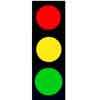### Traffic Lights

The game uses a 3x3 square board. 2 players take turns to play, either placing a red on an empty square, or changing a red to orange, or orange to green. The player who forms 3 of 1 colour in a line wins.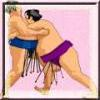### Sumo

A number game requiring a strategy.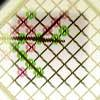### Line of Four

A game somewhat similar to 'noughts and crosses' on a much larger space.

# Flip Flop - Matching Cards

##### Age 5 to 11Challenge Level

We had many solutions come in from the Bristish Vietnamese International School after one of our NRICH team led some PD there. The following pupils sent in their thoughts, ideas and pictures of their results:

Khoa, Mai Khanh, Teppi, Nguyen Hoang Nam, Annie, Tuong Anh, Nguyá»…n, Phan Ngoc Thao Nhi, Huy Nam, Jonny, Mai, Hoang ha nguyen khanh, Quang Ha Nguon Khanh, Le Hoang Anh, Nguyen Minh Thanh An, Duc Thinh, Simon, Olivia, Michelle, Andy, Henry, Khang and Trá»‹nh.

Here are some of their observations:

My solution is if you found the first solution but it doesn't work out, the answer to the first solution may link to the next solution, so keep on looking for answer.

First I will choose two random squares if they do not match I will remember the number or the calculation, and also remember where they are. Next I will choose another random square. If it matches with one of the squares that I already chose then we already have a pair. But if they don't match just keep remembering the number or the calculation. Don't forget to remember where they are.

The method is trying to memorize the number or thinking fast math because this way will help you do questions more quickly. But if you want to do this you have to practise this frequently.

To solve the matching game with a faster time, you need to remember the place of the numbers and the addition or subtraction. The reason to do that is because there are a lot of numbers and calculations so we need to remember what number is correct for that calculation. For example, first time, you choose 12 and 11+3. Next time, you choose 14 and 7+5. So if you can remember the places of 12 and 11+3, you can make it 7+5 and 12, 11+3=14.

To be fast you must have somewhat of a good memory. You must remember where the equations are. First, calculate the equation and remember its position and answer so when you find the answer it will be easier. You can do the same to an answer, think of an equation that will most likely be in the game. If that doesn't work try to remember an equation that can make this answer. On my many game times, I discovered that most of the simple equations like 1+1+3 are most likely to pop up in the middle. You should explore the middle part first.

Here are some of the completed views they achieved: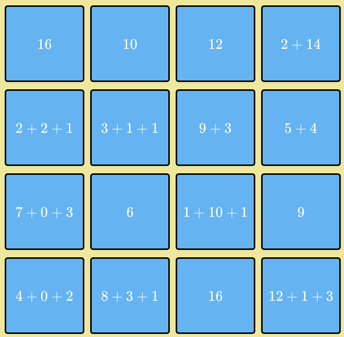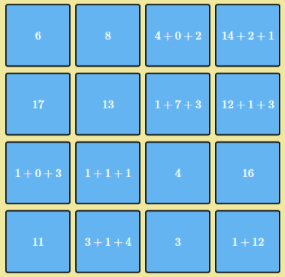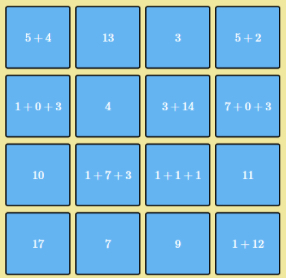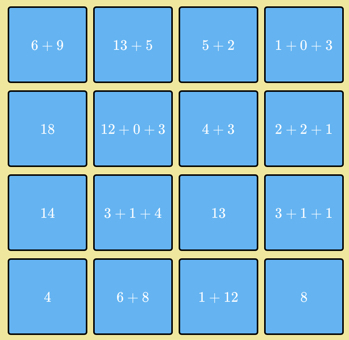Thanks all of you from the Bristish Vietnamese International School, well done!

We had the following submission from the International School of Brussels: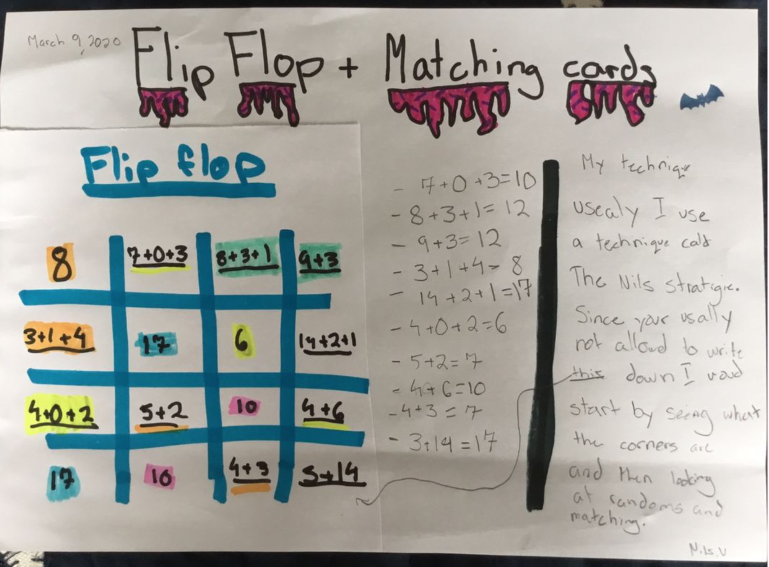Jeshan from Prince Edward Primary School wrote:

I chose numbers which add up to the number hidden on the card and then I solved four cards. I really enjoyed doing the whole game.

Thank you all for these submissions and comments.